Question

# The combustion of propane, C3H8, occurs via the reaction C3H8(g)+5O2(g)→3CO2(g)+4H2O(g) with heat of formation values given...

The combustion of propane, C3H8, occurs via the reaction C3H8(g)+5O2(g)→3CO2(g)+4H2O(g) with heat of formation values given by the following table:

Substance ΔH∘f (kJ/mol)

C3H8 (g)=  -104.7

CO2(g)= −393.5

H2O(g)= −241.8

Calculate the enthalpy for the combustion of 1 mole of propane.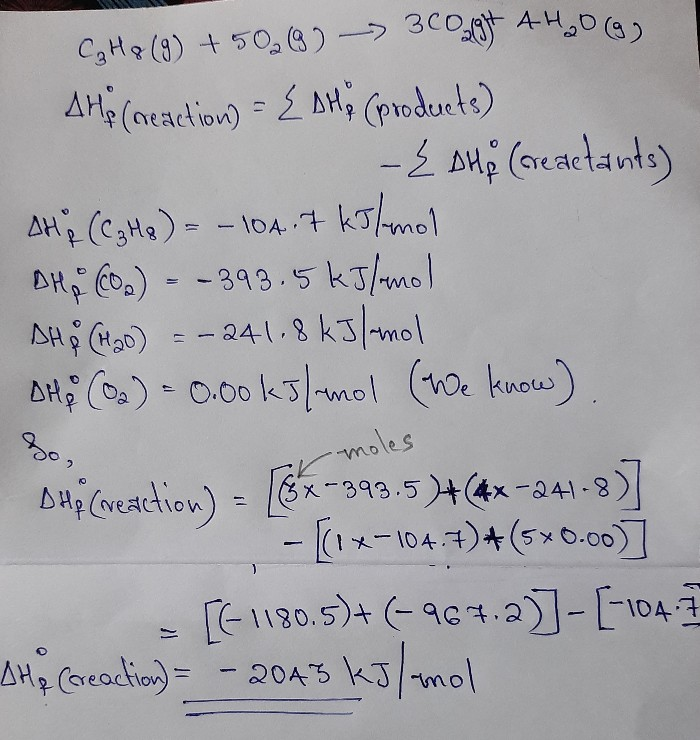#### Earn Coins

Coins can be redeemed for fabulous gifts.

Similar Homework Help Questions
• ### Question 3 Propane (C3H8) undergoes combustion according to the following thermochemical equation: C3H8(g) + 5 O2(g)...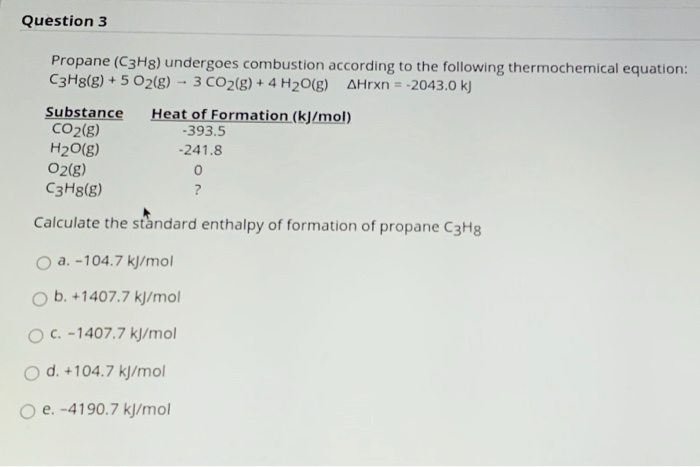Question 3 Propane (C3H8) undergoes combustion according to the following thermochemical equation: C3H8(g) + 5 O2(g) -- 3 CO2(g) + 4H2O(g) Arxn = -2043.0 kJ Substance Heat of Formation (kJ/mol) CO2(g) -393.5 H2O(g) -241.8 O2(g) 0 C3H8(g) ? Calculate the standard enthalpy of formation of propane C3H8 a. -104.7 kJ/mol ob. +1407.7 kJ/mol C. -1407.7 kJ/mol O d. +104.7 kJ/mol o e. -4190.7 kJ/mol

• ### Consider the combustion of propane: C3H8 (g) + 5O2 (g) → 3CO2 (g) + 4H2O(l) ΔH...

Consider the combustion of propane: C3H8 (g) + 5O2 (g) → 3CO2 (g) + 4H2O(l) ΔH = –2221 kJ Assume that all of the heat comes from the combustion of propane. Calculate ΔH in which 5.00 g of propane is burned in excess oxygen at constant pressure.

• ### The combustion of propane (C3H8) produces CO2 and H2O: C3H8 (g) + 5O2 (g) → 3CO2...

The combustion of propane (C3H8) produces CO2 and H2O: C3H8 (g) + 5O2 (g) → 3CO2 (g) + 4H2O (g) The reaction of 7.5 mol of O2 with 1.4 mol of C3H8 will produce ________ mol of CO2. Group of answer choices.

• ### calculate the enthalpy of the combustion of propane for your barbeque C3H8 + 5O2 -> 3CO2 + 4H2O propane -105 kJ...

calculate the enthalpy of the combustion of propane for your barbeque C3H8 + 5O2 -> 3CO2 + 4H2O propane -105 kJ/mol CO2 -394 kJ/mol Water -286 kJ/mol Ooxygen. 0

• ### The balanced chemical equation for the combustion of propane is C3H8(g) + 5O2(g) +3CO2(g) + 4H2O(g)...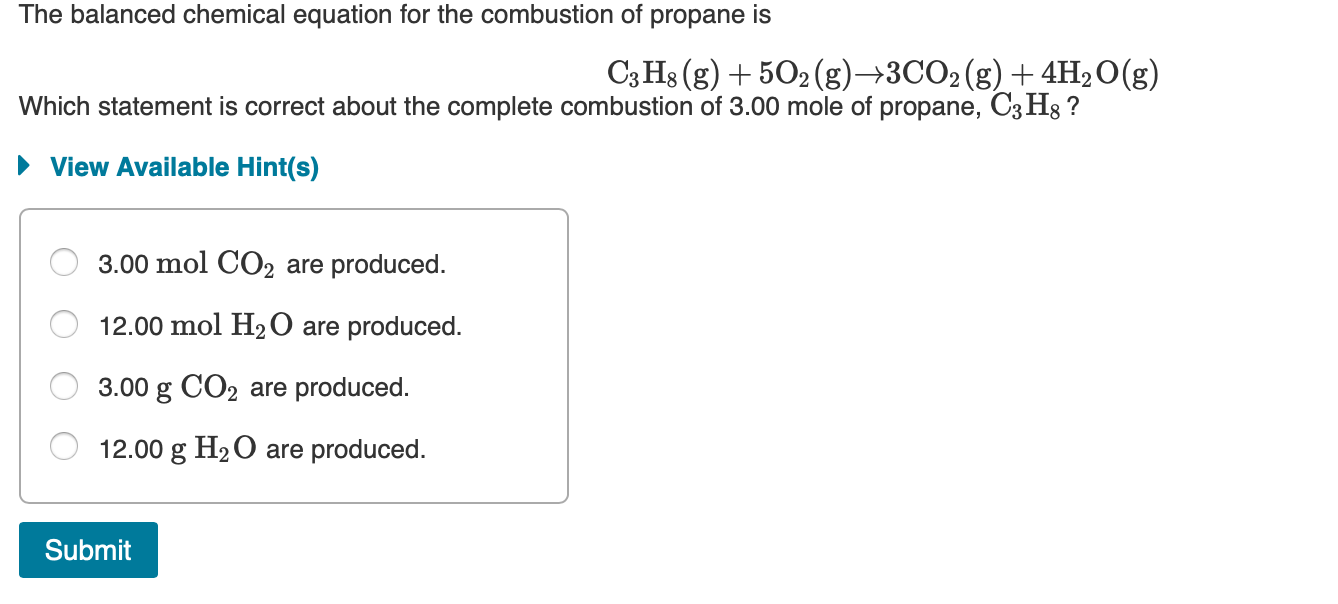The balanced chemical equation for the combustion of propane is C3H8(g) + 5O2(g) +3CO2(g) + 4H2O(g) Which statement is correct about the complete combustion of 3.00 mole of propane, C3Hg ? ► View Available Hint(s) O 3.00 mol CO2 are produced. O 12.00 mol H2O are produced. O 3.00 g CO2 are produced. O 12.00 g H2O are produced. Submit

• ### ﻿Propane (C3H8)burns according to the following balanced equation: C3H8(g)+5O2(g)→3CO2(g)+4H2O(g) Calculate ΔH∘rxnΔ for this reaction using standard...

﻿Propane (C3H8)burns according to the following balanced equation: C3H8(g)+5O2(g)→3CO2(g)+4H2O(g) Calculate ΔH∘rxnΔ for this reaction using standard enthalpies of formation. (The standard enthalpy of formation of gaseous propane is -103.9 kJ/molkJ/mol.) Express the enthalpy in kilojoules to four significant figures.

• ### Question 3 Propane (C3Hg) undergoes combustion according to the following thermochemical equation: C3H8(g) + 5 O2(g)...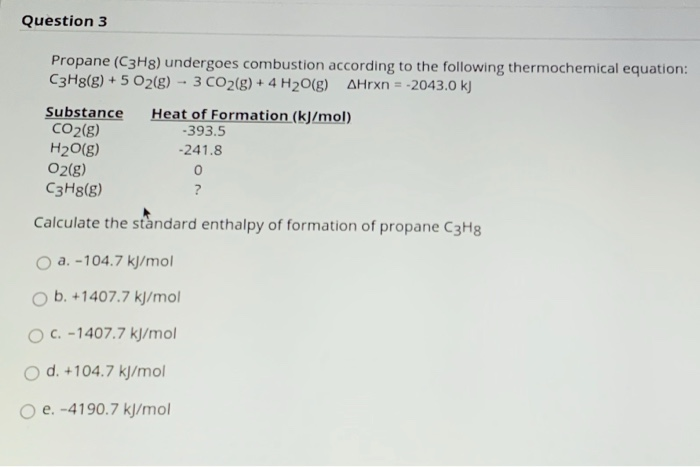Question 3 Propane (C3Hg) undergoes combustion according to the following thermochemical equation: C3H8(g) + 5 O2(g) - 3 CO2(g) + 4H2O(g) AHrxn=-2043.0 kJ Substance Heat of Formation (kJ/mol) CO2(g) -393.5 H2O(g) -241.8 O2(g) 0 C3H8(g) ? Calculate the standard enthalpy of formation of propane C3Hg a. -104.7 kJ/mol O b. +1407.7 kJ/mol O C. -1407.7 kJ/mol O d. +104.7 kJ/mol o e. -4190.7 kJ/mol uestion 4 Consider the evaporation of liquid water to water vapor at 125°C. What is true...

• ### The propane fuel (C3H8) used in gas barbeques burns according to this thermochemical equation. C3H8(g)+5O2(g)→3CO2(g)+4H2O(g)ΔH∘rxn=−2044kJ If...

The propane fuel (C3H8) used in gas barbeques burns according to this thermochemical equation. C3H8(g)+5O2(g)→3CO2(g)+4H2O(g)ΔH∘rxn=−2044kJ If a pork roast must absorb 1.7×103 kJ to fully cook, and if only 15 % of the heat produced by the barbeque is actually absorbed by the roast, what mass of CO2 is emitted into the atmosphere during the grilling of the pork roast?

• ### Given the following equation, C3H8 (g) + 5O2 (g) -> 3CO2 (g) + 4H2O (g); H comb = -2219 kJ/mole A) how much heat will be released when 10 g of propane is burned

Given the following equation, C3H8 (g) + 5O2 (g) -> 3CO2 (g) + 4H2O (g); H comb = -2219 kJ/mole A) how much heat will be released when 10 g of propane is burned? B) how much heat will be released when 10 g of water (H2O) is formed? Please show all work.

• ### The standard enthalpy change for the combustion of 1 mole of propane is -2043.0 kJ. CzH3(g)...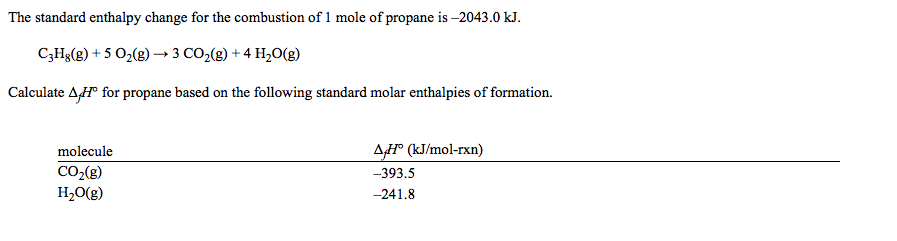The standard enthalpy change for the combustion of 1 mole of propane is -2043.0 kJ. CzH3(g) + 5 O2(g) + 3 CO2(g) + 4H2O(g) Calculate 4, Hº for propane based on the following standard molar enthalpies of formation. molecule CO2(g) H2O(g) 4,Hº (kJ/mol-rxn) -393.5 -241.8